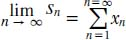# What does it mean if a series is convergent?

Convergence of a series is related to the convergence of a sequence, but don’t confuse them. The convergence of the sequence of partial sums (usually written as {sn}) differs greatly from the convergence of a sequence of numbers (usually written as {xn}). For example, the series Σxn (and its associated sequence of partial sums, or {sn}), is convergent if and only if the sequence {sn} is convergent. Thus, the total sum of the series is the limit of the sequence {sn}, seen as:Close

This is a web preview of the "The Handy Math Answer Book" app. Many features only work on your mobile device. If you like what you see, we hope you will consider buying. Get the App